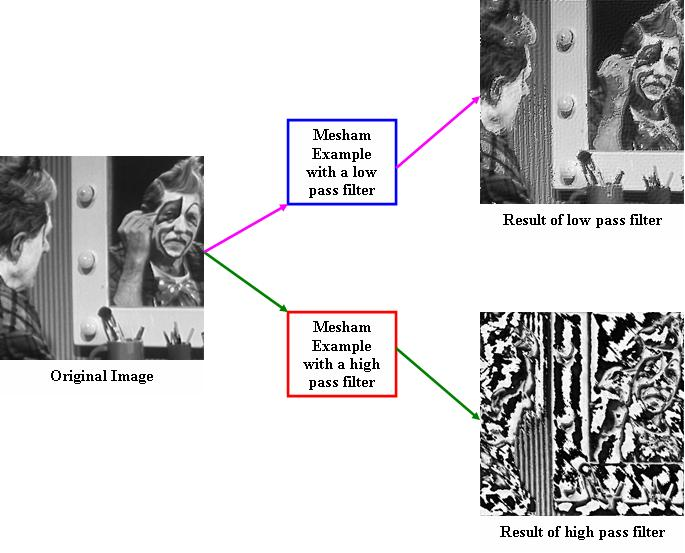# Image processing

Jump to navigationJump to search

## Overview

This example is one of the more complex examples we have written in the language. It allows the user to perform some parallel image processing on a black and white image. The image processing supported is applying a low or high pass filter on the image. However, to do this the image needs to be transformed into the frequency domain - and then requires transformation back into the time domain. At the core of the example is the FFT kernel, this is a basic Cooley Turkey FFT algorithm and there are more efficient ones out there. Having said that, the type information provided by the programmer allows the compiler to perform a large amount of optimisation during the translation process. By playing around you can change the filters and also invoke the high pass filter rather than the lowpass which the code does at the moment.## Performance

Performance of the Fast Fourier Transform (FFT) has been evaluated on a super computer cluster. Two different experiments were performed, one with an image size of 128MB and the other with an image size of 2GB. Evaluations were performed against the Fastest Fourier Transformation in the West (FFTW) and a book example for 128MB. As can be seen with an uneven distribution of data (10 and 20 processors) FFTW experiences severe slowdowns whereas the Mesham version does not (the compiler will optimise the code in this case to avoid any slow down.)

## Source Code

```#include <maths>
#include <io>
#include <string>

var n:=256; // image size
var m:=4; // number of processors
var filterThreshold:=10; // filtering threshold for high and low pass filters

function void main() {
var a:array[complex,n,n] :: allocated[single[on]];
var s:array[complex,n,n] :: allocated[horizontal[m] :: single[evendist]];
var s2:array[complex,n,n] :: allocated[horizontal[m] :: col[] :: single[evendist]];
var s3:array[complex,n,n] :: allocated[horizontal[m] :: single[evendist] :: share[s2]];
proc 0 {
loadfile("data/clown.ppm",a);
moveorigin(a);
};
s:=a;
var sinusiods:=computesin();
var p;
par p from 0 to m - 1 {
var i;
for i from s[p].low to s[p].high {
FFT(s[p][i-s[p].low],sinusiods);
};
};
s2:=s;
par p from 0 to m - 1 {
var i;
for i from s[p].low to s[p].high {
FFT(s3[p][i-s[p].low],sinusiods);
};
};
a:=s3;
proc 0 {
filter(a);
invert(a);
};

s:=a;
par p from 0 to m - 1 {
var i;
for i from s[p].low to s[p].high {
FFT(s[p][i-s[p].low],sinusiods);
};
};
s2:=s;
par p from 0 to m - 1 {
var i;
for i from s[p].low to s[p].high {
FFT(s3[p][i-s[p].low],sinusiods);
};
};
a:=s3;
proc 0 {
moveorigin(a);
descale(a);
writefile("newclown.ppm", a);
};
};

function array[complex] computesin() {
var elements:= n/2;
var sinusoid:array[complex, elements];
var j;
for j from 0 to (n / 2) - 1 {
var topass:Float;
topass:=((2 * pi() * j) / n);
sinusoid[j].i:=-sin(topass);
sinusoid[j].r:=cos(topass);
};
return sinusoid;
};

function Int getLogn() {
var logn:=0;
var nx:=n;
nx := nx >> 1;
while (nx >0) {
logn++;
nx := nx >> 1;
};
return logn;
};

function void moveorigin(var data: array[complex,n,n]) {
var i;
for i from 0 to n - 1 {
var j;
for j from 0 to n - 1 {
data[i][j].r:=data[i][j].r * pow(-1,(i + j));
data[i][j].i:=data[i][j].i * pow(-1,(i + j));
};
};
};

function void descale(var data: array[complex,n,n]) {
var i;
for i from 0 to n - 1 {
var j;
for j from 0 to n - 1 {
data[i][j].r:=data[i][j].r / (n * n) ;
data[i][j].i:=-(data[i][j].i / (n * n));
};
};
};

function void invert(var data : array[complex,n,n]) {
var i;
for i from 0 to n - 1 {
var j;
for j from 0 to n - 1 {
data[i][j].i:=-data[i][j].i;
};
};
};

function void FFT(var data : array[complex,n], var sinusoid:array[complex]) {
var i2:=getLogn();
bitreverse(data); // data decomposition
var f0:Double;
var f1:Double;
var increvec;
for increvec from 2 to n {
i2:=i2 - 1;
var i0;
for i0 from 0 to ((increvec / 2) - 1)  {
// below computes the sinusoid for this spectra
var i1;
for i1 from 0 to n - 1 {
// do butterfly for each point in the spectra
f0:=(data[i0 + i1 + (increvec / 2)].r * sinusoid[i0 << i2].r)- (data[i0 + i1 + (increvec / 2)].i * sinusoid[i0 << i2].i);
f1:=(data[i0 + i1 + (increvec / 2)].r * sinusoid[i0 << i2].i)+ (data[i0 + i1 + (increvec / 2)].i * sinusoid[i0 << i2].r);

data[i0 + i1 + (increvec / 2)].r:= data[i0 + i1].r- f0;
data[i0 + i1 + (increvec / 2)].i:=data[i0 + i1].i - f1;
data[i0 + i1].r := data[i0 + i1].r + f0;
data[i0 + i1].i := data[i0 + i1].i + f1;

i1:=(i1 + increvec) - 1;
};
};
increvec:=(increvec * 2) - 1;
};
};

function void loadfile(var name:String,var data:array[complex,n,n]) {
var file:=open(name,"r");
readline(file);
readline(file);
readline(file);
readline(file);
var i;
for i from 0 to n - 1 {
var j;
for j from 0 to n - 1 {
var red:=readchar(file);
readchar(file);readchar(file);
data[i][j].r:=red;
data[i][j].i:=red;
};
};
close(file);
};

function void writefile(var thename:String, var data:array[complex,n,n]) {
var file:=open(thename,"w");
writestring(file,"P6\n# CREATOR: LOGS Program\n");
writestring(file,itostring(n));
writestring(file," ");
writestring(file,itostring(n));
writestring(file,"\n255\n");
var i;
for i from 0 to n - 1 {
var j;
for j from 0 to n - 1 {
writebinary(file,data[i][j].r);
writebinary(file,data[i][j].r);
writebinary(file,data[i][j].r);
};
};
close(file);
};

function Int lowpass(var i:Int, var j:Int) {
var val:=sqr(i) + sqr(j);
if (sqrt(val) < filterThreshold) return 1;
return 0;
};

function Int highpass(var i:Int, var j:Int) {
var val:=sqr(i) + sqr(j);
if (sqrt(val) > (255-filterThreshold)) return 1;
return 0;
};

function void filter(var data: array[complex,n,n]) {
var i;
for i from 0 to n - 1 {
var j;
for j from 0 to n - 1 {
data[i][j].r:=data[i][j].r * lowpass(i,j) * highpass(i,j);
data[i][j].i:=data[i][j].i * lowpass(i,j) * highpass(i,j);
};
};
};

function void bitreverse(var a:array[complex,n]) {
var j:=0;
var k:Int;
var i;
for i from 0 to n-2 {
if (i < j) {
var swap_temp:Double;
swap_temp:=a[j].r;
a[j].r:=a[i].r;
a[i].r:=swap_temp;
swap_temp:=a[j].i;
a[j].i:=a[i].i;
a[i].i:=swap_temp;
};
k := n >> 1;
while (k <= j) {
j := j - k;
k := k >>1;
};
j := j + k;
};
};
```

This version requires at least Mesham version 1.0